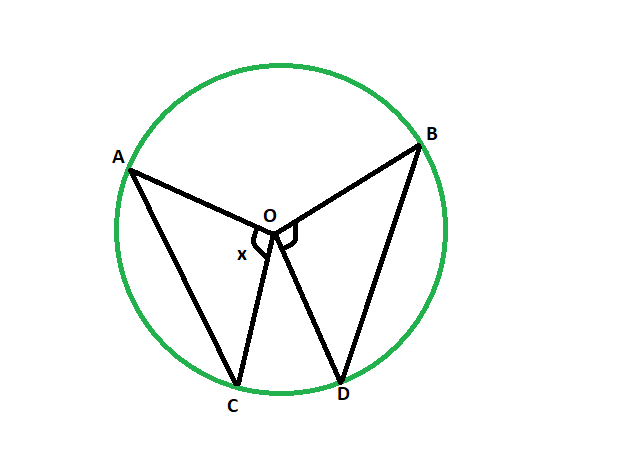# Angle subtended by the chord when the angle subtended by another chord of same length is given

• Last Updated : 30 May, 2022

Given a circle having two chords of equal length. The angle subtended by one of the chords to the centre is given. The task here is to find the measure of the angle subtended by another chord at the centre.
Examples:

```Input: 48
Output: 48 degrees

Input: 82
Output: 82 degrees```Approach
Let AC & BD are the two equal chords of the circle having center at O.let the angle subtended by the chord AC be x degrees.
now,
in triangle AOC & BOD
AO = OB(radii of same circle)
AB = CD(equal chords)
OC = OD(radii of same circle)
so, the triangles AOC & BOD are congruent to each other
so, angle AOC = angle BOD

Equal chords of a circle subtend equal angles at the centre.

Below is the implementation of the above approach:

## C++

 `// C++ program to find the angle` `// subtended at the center by the chord` `// when the angle subtended at center` `// by another chord of equal length is given`   `#include ` `using` `namespace` `std;`   `void` `angleequichord(``int` `z)` `{` `    ``cout << ``"The angle subtended at the center is "` `         ``<< z << ``" degrees"` `<< endl;` `}`   `// Driver code` `int` `main()` `{` `    ``int` `z = 48;` `    ``angleequichord(z);` `    ``return` `0;` `}`

## Java

 `// Java program to find the angle` `// subtended at the center by the chord` `// when the angle subtended at center` `// by another chord of equal length is given`   `import` `java.io.*;`   `class` `GFG ` `{` `    `  `static` `void` `angleequichord(``int` `z)` `{` `    ``System.out.println ( ``"The angle subtended at the center is "``+` `        ``z + ``" degrees"``);` `}`   `// Driver code` `public` `static` `void` `main (String[] args) ` `{`   `    ``int` `z = ``48``;` `    ``angleequichord(z);` `}` `}`   `// This code is contributed by ajit.`

## Python3

 `# Python3 program to find the angle ` `# subtended at the center by the chord ` `# when the angle subtended at center ` `# by another chord of equal length is given `   `def` `angleequichord(z) : `   `    ``print``(``"The angle subtended at"``, ` `          ``"the center is"``, z ,``"degrees"` `);`   `# Driver code ` `if` `__name__ ``=``=` `"__main__"` `: ` `    `  `    ``z ``=` `48``; ` `    ``angleequichord(z); ` `    `  `# This code is contributed by AnkitRai01`

## C#

 `// C# program to find the angle` `// subtended at the center by the chord` `// when the angle subtended at center` `// by another chord of equal length is given` `using` `System;` `    `  `class` `GFG ` `{` `    `  `static` `void` `angleequichord(``int` `z)` `{` `    ``Console.WriteLine( ``"The angle subtended at the center is "``+` `        ``z + ``" degrees"``);` `}`   `// Driver code` `public` `static` `void` `Main (String[] args) ` `{`   `    ``int` `z = 48;` `    ``angleequichord(z);` `}` `}`   `/* This code contributed by PrinciRaj1992 */`

## Javascript

 ``

Output:

`The angle subtended at the center is 48 degrees`

Time Complexity: O(1)

Auxiliary Space: O(1)

My Personal Notes arrow_drop_up
Recommended Articles
Page :